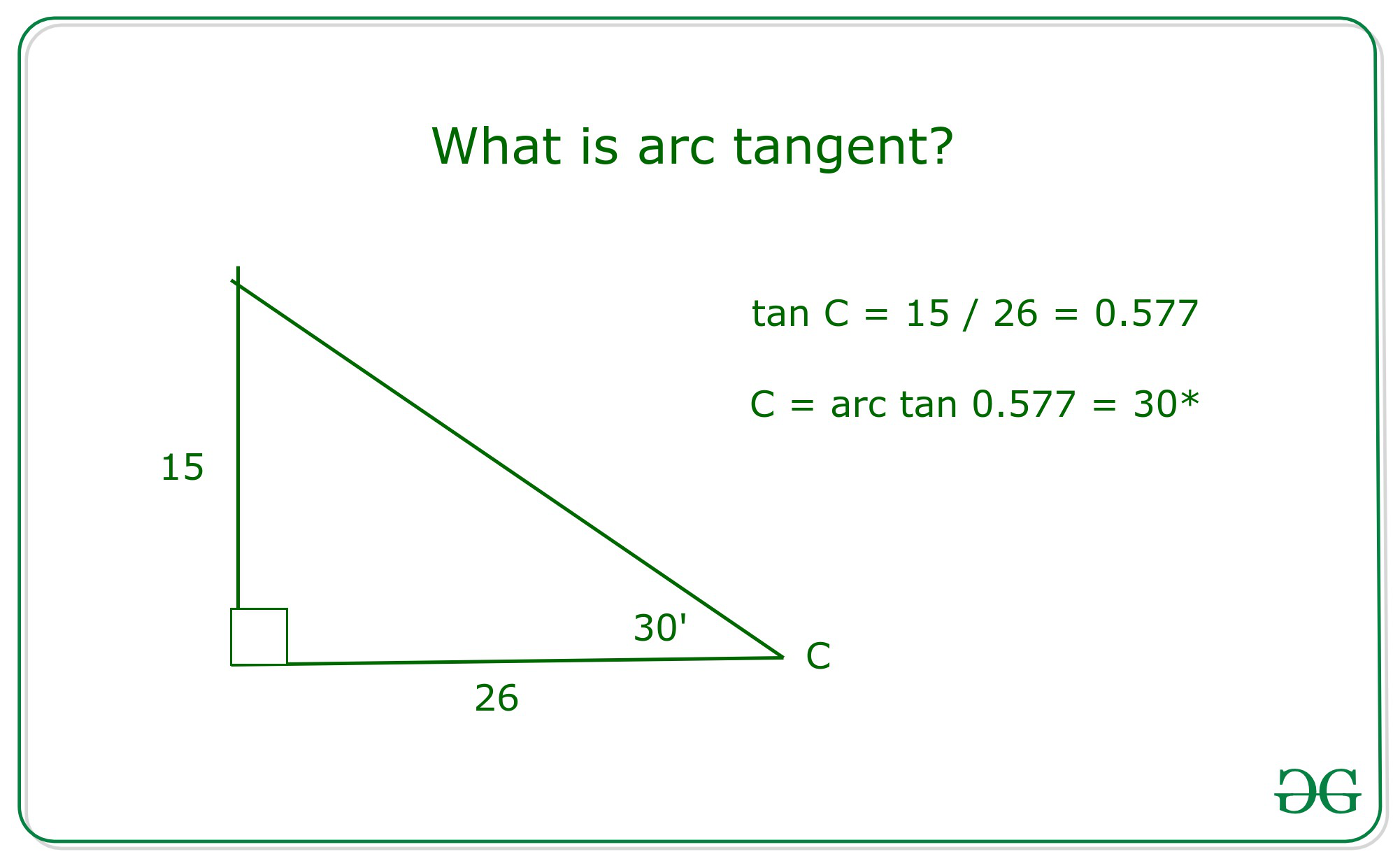Related Articles
How to find arctangent with Examples
• Last Updated : 21 Oct, 2020

### What is arc tangent?

The arctangent is the inverse of the tangent function. It returns the angle whose tangent is the given number.catan() is an inbuilt function in <complex.h> header file which returns the complex inverse tangent (or arc tangent) of any constant, which divides the imaginary axis on the basis of the inverse tangent in the closed interval [-i, +i] (where i stands for iota), used for evaluation of a complex object say z is on imaginary axis whereas to determine a complex object which is real or integer, then internally invokes pre-defined methods as:

Syntax:

```atan(double arg);
atanf(float arg);
atanl(long double arg);
where arg is a floating-point value

catan(double complex z);
catanf(float complex z);
catanl( long double complex z);
where z is a Type – generic macro
```

Parameter: These functions accept one mandatory parameter z which specifies the inverse tangent. The parameter can be of double, float, or long double datatype.

Return Value: This function returns complex arc tangent/arc tangent according to the type of the argument passed.

Below are the programs illustrate the above method:

Program 1: This program will illustrate the functions atan(), atanf(), and atanl() computes the principal value of the arc tangent of floating – point argument. If a range error occurs due to underflow, the correct result after rounding off is returned.

## C

 `// C program to illustrate the use``// of functions atan(), atanf(),``// and atanl()``#include ``#include `` ` `// Driver Code``int` `main()``{``    ``// For function atan()``    ``printf``(``"atan(1) = %lf, "``,``           ``atan``(1));``    ``printf``(``" 4*atan(1)=%lf\n"``,``           ``4 * ``atan``(1));`` ` `    ``printf``(``"atan(-0.0) = %+lf, "``,``           ``atan``(-0.0));``    ``printf``(``"atan(+0.0) = %+lf\n"``,``           ``atan``(0));`` ` `    ``// For special values INFINITY``    ``printf``(``"atan(Inf) = %lf, "``,``           ``atan``(INFINITY));``    ``printf``(``"2*atan(Inf) = %lf\n\n"``,``           ``2 * ``atan``(INFINITY));`` ` `    ``// For function atanf()``    ``printf``(``"atanf(1.1) = %f, "``,``           ``atanf(1.1));``    ``printf``(``"4*atanf(1.5)=%f\n"``,``           ``4 * atanf(1.5));`` ` `    ``printf``(``"atanf(-0.3) = %+f, "``,``           ``atanf(-0.3));``    ``printf``(``"atanf(+0.3) = %+f\n"``,``           ``atanf(0.3));`` ` `    ``// For special values INFINITY``    ``printf``(``"atanf(Inf) = %f, "``,``           ``atanf(INFINITY));``    ``printf``(``"2*atanf(Inf) = %f\n\n"``,``           ``2 * atanf(INFINITY));`` ` `    ``// For function atanl()``    ``printf``(``"atanl(1.1) = %Lf, "``,``           ``atanl(1.1));``    ``printf``(``"4*atanl(1.7)=%Lf\n"``,``           ``4 * atanl(1.7));`` ` `    ``printf``(``"atanl(-1.3) = %+Lf, "``,``           ``atanl(-1.3));``    ``printf``(``"atanl(+0.3) = %+Lf\n"``,``           ``atanl(0.3));`` ` `    ``// For special values INFINITY``    ``printf``(``"atanl(Inf) = %Lf, "``,``           ``atanl(INFINITY));``    ``printf``(``"2*atanl(Inf) = %Lf\n\n"``,``           ``2 * atanl(INFINITY));`` ` `    ``return` `0;``}`
Output:
```atan(1) = 0.785398,  4*atan(1)=3.141593
atan(-0.0) = -0.000000, atan(+0.0) = +0.000000
atan(Inf) = 1.570796, 2*atan(Inf) = 3.141593

atanf(1.1) = 0.832981, 4*atanf(1.5)=3.931175
atanf(-0.3) = -0.291457, atanf(+0.3) = +0.291457
atanf(Inf) = 1.570796, 2*atanf(Inf) = 3.141593

atanl(1.1) = 0.832981, 4*atanl(1.7)=4.156289
atanl(-1.3) = -0.915101, atanl(+0.3) = +0.291457
atanl(Inf) = 1.570796, 2*atanl(Inf) = 3.141593
```

Program 2: This program will illustrate the functions catan(), catanf(), and catanl() computes the principal value of the arc tangent of complex number as argument.

## C

 `// C program to illustrate the use``// of functions catan(), catanf(),``// and catanl()``#include ``#include ``#include `` ` `// Driver Code``int` `main()``{``    ``// Given Complex Number``    ``double` `complex z1 = catan(2 * I);`` ` `    ``// Function catan()``    ``printf``(``"catan(+0 + 2i) = %lf + %lfi\n"``,``           ``creal(z1), cimag(z1));`` ` `    ``// Complex(0, + INFINITY)``    ``double` `complex z2 = 2``                        ``* catan(2 * I * DBL_MAX);``    ``printf``(``"2*catan(+0 + i*Inf) = %lf%+lfi\n"``,``           ``creal(z2), cimag(z2));`` ` `    ``printf``(``"\n"``);`` ` `    ``// Function catanf()``    ``float` `complex z3 = catanf(2 * I);``    ``printf``(``"catanf(+0 + 2i) = %f + %fi\n"``,``           ``crealf(z3), cimagf(z3));`` ` `    ``// Complex(0, + INFINITY)``    ``float` `complex z4 = 2``                       ``* catanf(2 * I * DBL_MAX);``    ``printf``(``"2*catanf(+0 + i*Inf) = %f + %fi\n"``,``           ``crealf(z4), cimagf(z4));`` ` `    ``printf``(``"\n"``);`` ` `    ``// Function catanl()``    ``long` `double` `complex z5 = catanl(2 * I);``    ``printf``(``"catan(+0+2i) = %Lf%+Lfi\n"``,``           ``creall(z5), cimagl(z5));`` ` `    ``// Complex(0, + INFINITY)``    ``long` `double` `complex z6 = 2``                             ``* catanl(2 * I * DBL_MAX);``    ``printf``(``"2*catanl(+0 + i*Inf) = %Lf + %Lfi\n"``,``           ``creall(z6), cimagl(z6));``}`
Output:
```catan(+0 + 2i) = 1.570796 + 0.549306i
2*catan(+0 + i*Inf) = 3.141593+0.000000i

catanf(+0 + 2i) = 1.570796 + 0.549306i
2*catanf(+0 + i*Inf) = 3.141593 + 0.000000i

catan(+0+2i) = 1.570796+0.549306i
2*catanl(+0 + i*Inf) = 3.141593 + 0.000000i
```

Program 3: This program will illustrate the functions catanh(), catanhf(), and catanhl() computes the complex arc hyperbolic tangent of z along the real axis and in the interval [-i*PI/2, +i*PI/2] along the imaginary axis.

## C

 `// C program to illustrate the use``// of functions  catanh(), catanhf(),``// and catanhl()``#include ``#include `` ` `// Driver Code``int` `main()``{``    ``// Function catanh()``    ``double` `complex z1 = catanh(2);``    ``printf``(``"catanh(+2+0i) = %lf%+lfi\n"``,``           ``creal(z1), cimag(z1));`` ` `    ``// for any z, atanh(z) = atan(iz)/i``    ``// I denotes Imaginary``    ``// part of the complex number``    ``double` `complex z2 = catanh(1 + 2 * I);``    ``printf``(``"catanh(1+2i) = %lf%+lfi\n\n"``,``           ``creal(z2), cimag(z2));`` ` `    ``// Function catanhf()``    ``float` `complex z3 = catanhf(2);``    ``printf``(``"catanhf(+2+0i) = %f%+fi\n"``,``           ``crealf(z3), cimagf(z3));`` ` `    ``// for any z, atanh(z) = atan(iz)/i``    ``float` `complex z4 = catanhf(1 + 2 * I);``    ``printf``(``"catanhf(1+2i) = %f%+fi\n\n"``,``           ``crealf(z4), cimagf(z4));`` ` `    ``// Function catanh()``    ``long` `double` `complex z5 = catanhl(2);``    ``printf``(``"catanhl(+2+0i) = %Lf%+Lfi\n"``,``           ``creall(z5), cimagl(z5));`` ` `    ``// for any z, atanh(z) = atan(iz)/i``    ``long` `double` `complex z6 = catanhl(1 + 2 * I);``    ``printf``(``"catanhl(1+2i) = %Lf%+Lfi\n\n"``,``           ``creall(z6), cimagl(z6));``}`
Output:
```catanh(+2+0i) = 0.549306+1.570796i
catanh(1+2i) = 0.173287+1.178097i

catanhf(+2+0i) = 0.549306+1.570796i
catanhf(1+2i) = 0.173287+1.178097i

catanhl(+2+0i) = 0.549306+1.570796i
catanhl(1+2i) = 0.173287+1.178097i
```

Want to learn from the best curated videos and practice problems, check out the C Foundation Course for Basic to Advanced C.

My Personal Notes arrow_drop_up